# Test: Partial Derivatives, Gradient- 1

Test Description

## 20 Questions MCQ Test GATE Mechanical (ME) 2023 Mock Test Series | Test: Partial Derivatives, Gradient- 1

Test: Partial Derivatives, Gradient- 1 for Civil Engineering (CE) 2023 is part of GATE Mechanical (ME) 2023 Mock Test Series preparation. The Test: Partial Derivatives, Gradient- 1 questions and answers have been prepared according to the Civil Engineering (CE) exam syllabus.The Test: Partial Derivatives, Gradient- 1 MCQs are made for Civil Engineering (CE) 2023 Exam. Find important definitions, questions, notes, meanings, examples, exercises, MCQs and online tests for Test: Partial Derivatives, Gradient- 1 below.
Solutions of Test: Partial Derivatives, Gradient- 1 questions in English are available as part of our GATE Mechanical (ME) 2023 Mock Test Series for Civil Engineering (CE) & Test: Partial Derivatives, Gradient- 1 solutions in Hindi for GATE Mechanical (ME) 2023 Mock Test Series course. Download more important topics, notes, lectures and mock test series for Civil Engineering (CE) Exam by signing up for free. Attempt Test: Partial Derivatives, Gradient- 1 | 20 questions in 60 minutes | Mock test for Civil Engineering (CE) preparation | Free important questions MCQ to study GATE Mechanical (ME) 2023 Mock Test Series for Civil Engineering (CE) Exam | Download free PDF with solutions
 1 Crore+ students have signed up on EduRev. Have you?
Test: Partial Derivatives, Gradient- 1 - Question 1

### Consider the function f(x) = x2 – x – 2. The maximum value of f(x) in the closed interval [–4, 4] is

Detailed Solution for Test: Partial Derivatives, Gradient- 1 - Question 1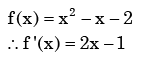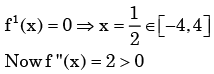∴ f (x )has minimum at x= 1 / 2 It Shows that a maximum value that will be at x = 4  or x = - 4

At x = 4, f (x )= 10

∴ At x= −4, f (x ) = 18

∴ At x= −4, f (x ) has a maximum.

Test: Partial Derivatives, Gradient- 1 - Question 2

###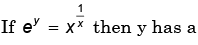Detailed Solution for Test: Partial Derivatives, Gradient- 1 - Question 2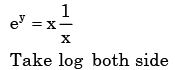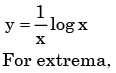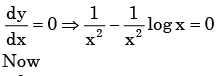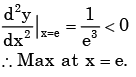Test: Partial Derivatives, Gradient- 1 - Question 3

###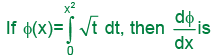Detailed Solution for Test: Partial Derivatives, Gradient- 1 - Question 3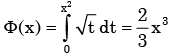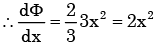Test: Partial Derivatives, Gradient- 1 - Question 4

The function f(x) = x3- 6x2+ 9x+25 has

Detailed Solution for Test: Partial Derivatives, Gradient- 1 - Question 4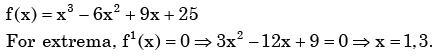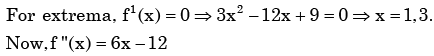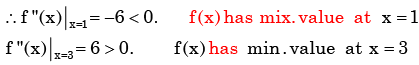Test: Partial Derivatives, Gradient- 1 - Question 5

The function f(x,y) = 2x2 +2xy – y3 has

Detailed Solution for Test: Partial Derivatives, Gradient- 1 - Question 5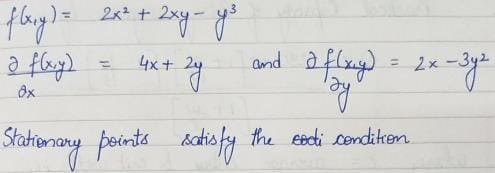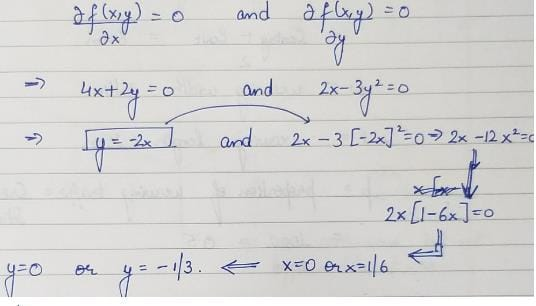Test: Partial Derivatives, Gradient- 1 - Question 6

Equation of the line normal to function f(x) = (x-8)2/3+1 at P(0,5) is

Detailed Solution for Test: Partial Derivatives, Gradient- 1 - Question 6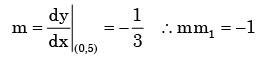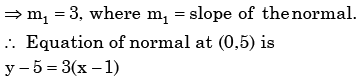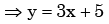Test: Partial Derivatives, Gradient- 1 - Question 7

The distance between the origin and the point nearest to it on the surface z2 = 1 + xy is

Detailed Solution for Test: Partial Derivatives, Gradient- 1 - Question 7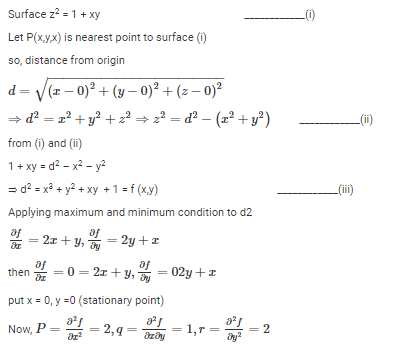or pr – q2 = 4 – 1 = 3 > 0 and r = +ve

so f(xy) is minimum at (0,0)

Hence, minimum value of d2 at (0,0)

d2 = x2 + y2 + xy + 1 = (0)2 + (0)2 + (0)(0) + 1 = 1

Then the nearest point is

z2 = 1 + xy = 1+ (0)(0) = 1

or z = 1

Test: Partial Derivatives, Gradient- 1 - Question 8

Given a function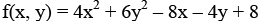The optimal value of f(x, y)

Detailed Solution for Test: Partial Derivatives, Gradient- 1 - Question 8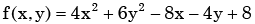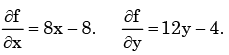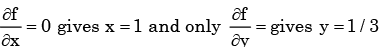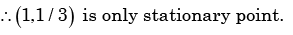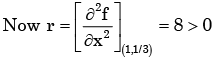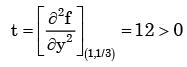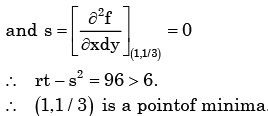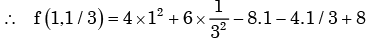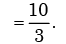Test: Partial Derivatives, Gradient- 1 - Question 9

For the function f(x) = x2e-x, the maximum occurs when x is equal to

Detailed Solution for Test: Partial Derivatives, Gradient- 1 - Question 9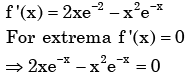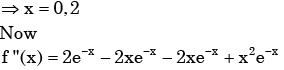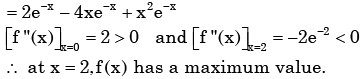Test: Partial Derivatives, Gradient- 1 - Question 10

A cubic polynomial with real coefficients

Detailed Solution for Test: Partial Derivatives, Gradient- 1 - Question 10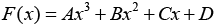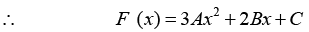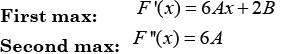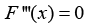So maximum two extrema and three zero crossing

Test: Partial Derivatives, Gradient- 1 - Question 11

Given y = x2 + 2x + 10, the value of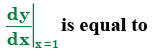Detailed Solution for Test: Partial Derivatives, Gradient- 1 - Question 11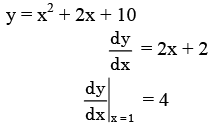Test: Partial Derivatives, Gradient- 1 - Question 12

Consider the function y = x2 – 6x + 9. The maximum value of y obtained when x varies over the interval 2 to 5 is

Detailed Solution for Test: Partial Derivatives, Gradient- 1 - Question 12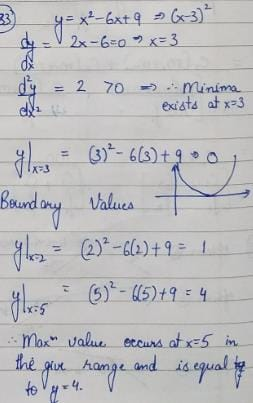Test: Partial Derivatives, Gradient- 1 - Question 13

Consider the function y = x2 – 6x + 9. The maximum value of y obtained when x varies over the interval 2 to 5 is

Detailed Solution for Test: Partial Derivatives, Gradient- 1 - Question 13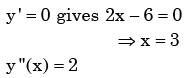Test: Partial Derivatives, Gradient- 1 - Question 14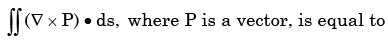Test: Partial Derivatives, Gradient- 1 - Question 15

The magnitude of the gradient of the function f = xyz3 at (1,0,2) is

Detailed Solution for Test: Partial Derivatives, Gradient- 1 - Question 15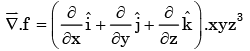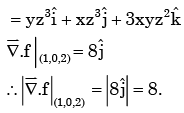Test: Partial Derivatives, Gradient- 1 - Question 16

The expression curl (grad f), where f is a scalar function, is

Detailed Solution for Test: Partial Derivatives, Gradient- 1 - Question 16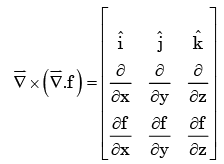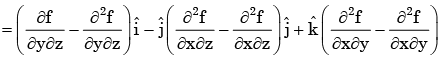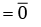Test: Partial Derivatives, Gradient- 1 - Question 17

If the velocity vector in a two – dimensional flow field is given by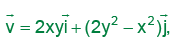the  vorticity vector, curl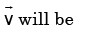Detailed Solution for Test: Partial Derivatives, Gradient- 1 - Question 17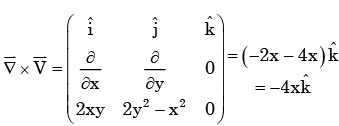Test: Partial Derivatives, Gradient- 1 - Question 18

The vector field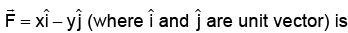Detailed Solution for Test: Partial Derivatives, Gradient- 1 - Question 18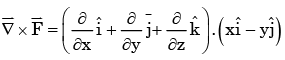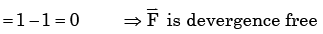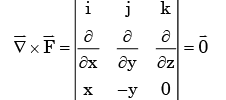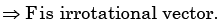Test: Partial Derivatives, Gradient- 1 - Question 19

The angle between two unit-magnitude co-planar vectors P (0.866, 0.500, 0) and Q (0.259, 0.966, 0) will be

Detailed Solution for Test: Partial Derivatives, Gradient- 1 - Question 19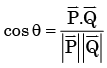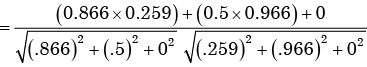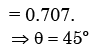Test: Partial Derivatives, Gradient- 1 - Question 20

Stokes theorem connects

## GATE Mechanical (ME) 2023 Mock Test Series

27 docs|243 tests
 Use Code STAYHOME200 and get INR 200 additional OFF Use Coupon Code